Students can download Maths Chapter 4 Geometry Ex 4.5 Questions and Answers, Notes, Samacheer Kalvi 9th Maths Guide Pdf helps you to revise the complete Tamilnadu State Board New Syllabus, helps students complete homework assignments and to score high marks in board exams.

## Tamilnadu Samacheer Kalvi 9th Maths Solutions Chapter 4 Geometry Ex 4.5

Question 1.
Construct the ΔLMN such that LM = 7.5 cm, MN = 5cm and LN = 8cm. Locate its centroid.
Solution: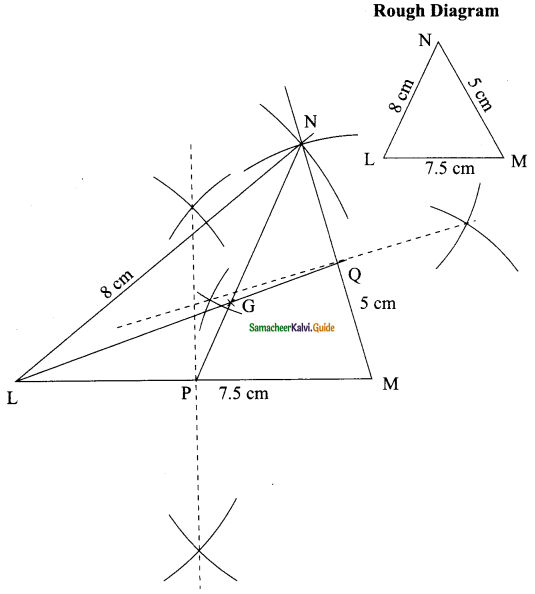Steps for construction:
Step 1: Draw the ΔLMN using the given measurement LM = 7.5 cm, MN = 5cm and LN = 8cm.
Step 2: Construct the perpendicular bisectors of any two sides LM and MN intersect LM at P and MN at Q respectively.
Step 3: Draw the median LQ and PN meet at G.
The point G is the centroid of the given ΔLMN.Question 2.
Draw and locate the centroid of the triangle ABC where right angle at A, AB = 4cm and AC = 3 cm.
Solution: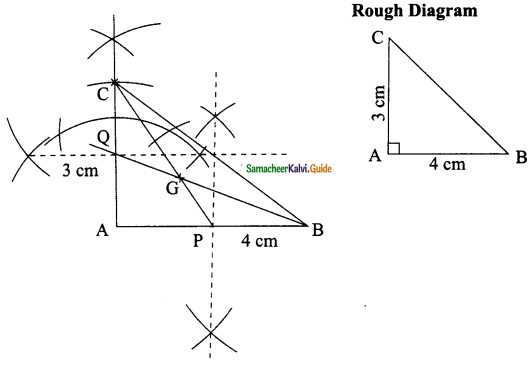Steps for construction:
Step 1: Draw the ΔABC using the given measurement AB = 4cm and AC = 3 cm and ZA = 90°.
Step 2: Construct the perpendicular bisectors of any two sides AB and AC to find the mid-points P and Q of AB and AC.
Step 3: Draw the medians PC and BQ intersect at G.
The point G is the centroid of the given ΔABC.Question 3.
Draw the ΔABC, where AB = 6cm, ∠B = 110° and AC = 9cm and construct the centroid.
Solution: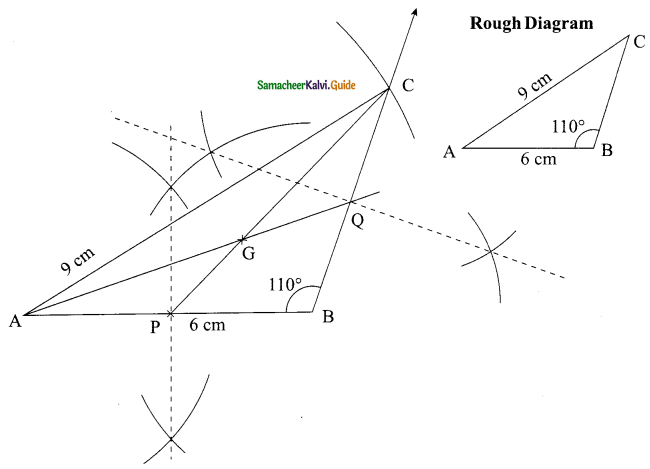Steps for construction:
Step 1: Draw the ΔABC using the given measurement AB = 6cm, AC = 9cm and ∠B =110°.
Step 2: Construct the perpendicular bisectors of any two sides AB and BC to find the mid-points P and Q of AB and BC.
Step 3: Draw the medians PC and AQ intersect at G.
The point G is the centroid of the given ΔABC.Question 4.
Construct the ΔPQR such that PQ = 5cm, PR = 6cm and ∠QPR = 60° and locate its centroid.
Solution: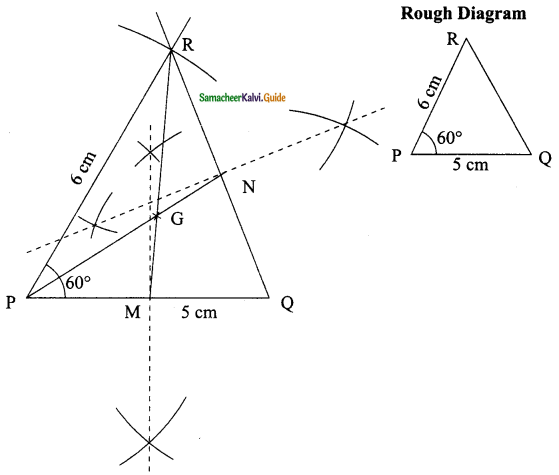Steps for construction:
Step 1 : Draw ΔPQR using the given measurements PQ = 5cm, PR = 6cm and ∠P = 60°.
Step 2 : Construct the perpendicular bisectors of any two sides PQ and QR to find the mid-points of M and N respectively.
Step 3 : Draw the median PN and MR and let them meet at G.
The point G is the centroid of the given ΔPQR.Question 5.
Draw ΔPQR with sides PQ = 7 cm, QR = 8 cm and PR = 5 cm and construct its Orthocentre.
Solution: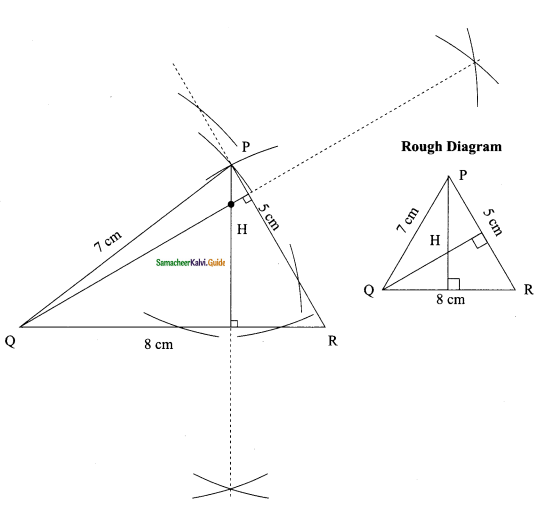Steps for construction:
Step 1: Draw the ΔPQR with the given measurements.
Step 2: Construct altitudes from any two vertices P and Q to their opposite sides QR and PR respectively.
Step 3: The point of intersection of the altitude H is the orthocentre of the given ΔPQR.Question 6.
Draw an equilateral triangle of sides 6.5 cm and locate its Orthocentre.
Solution: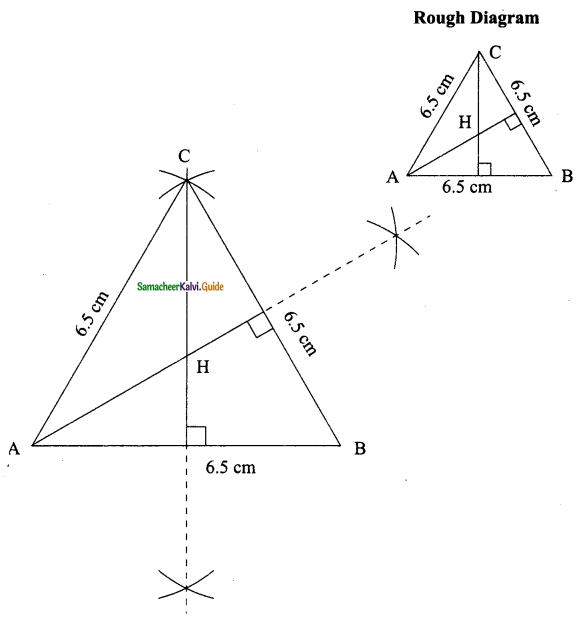Steps for construction:
Step 1: Draw the ΔABC with the given measurements.
Step 2: Construct altitudes from any two vertices A and C to their opposite sides BC and AB respectively.
Step 3: The point of intersection of the altitude H is the orthocentre of the given ΔABC.Question 7.
Draw ΔABC, where AB = 6 cm, ∠B = 110° and BC = 5 cm and construct its Orthocentre.
Solution: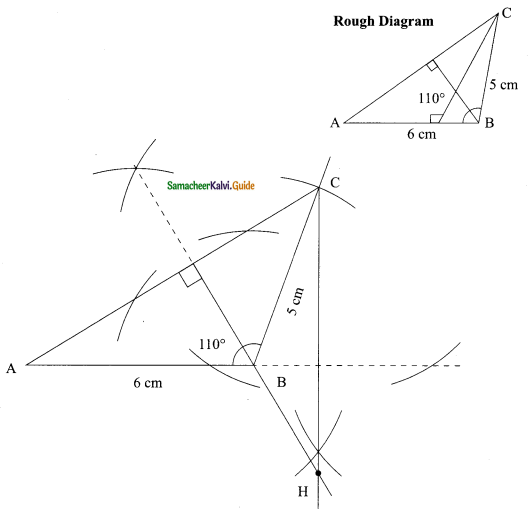Steps for construction:
Step 1: Draw the ΔABC with the given measurements.
Step 2: Construct altitudes from any two vertices B and C to their opposite sides AC and BC respectively.
Step 3: The point of intersection of the altitude H is the orthocentre of the given ΔABC.Question 8.
Draw and locate the Orthocentre of a right triangle PQR where PQ = 4.5 cm, QR = 6 cm and PR = 7.5 cm.
Solution: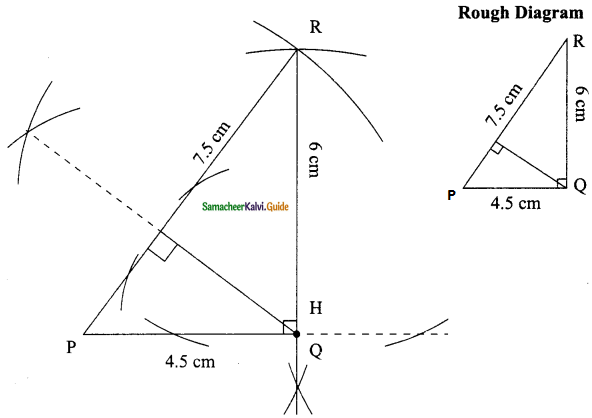Steps for construction:
Step 1: Draw the ΔPQR with the given measures.
Step 2: Construct altitude from any two vertices Q and R to their opposite side PR and PQ respectively.
Step 3: The point of intersection of the altitude H is the orthocentre of the given ΔPQR.